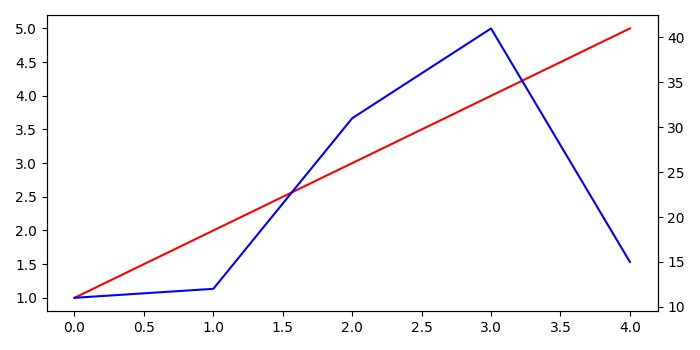# How do I plot multiple X or Y axes in Matplotlib?

To plot multiple X or Y axis, we can use twinx() or twiny() methods, we can take the following Steps −

• Using subplots() method, create a figure and a set of subplots.

• Plot [1, 2, 3, 4, 5] data points on the left Y-axis scales.

• Using twinx() method, create a twin of Axes with a shared X-axis but independent Y-axis, ax2.

• Plot [11, 12, 31, 41, 15] data points on the right Y-axis scale, with blue color.

• Using tight_layout() method, adjust the padding between and around the subplots.

• To display the figure, use show() method.

## Example

import matplotlib.pyplot as plt
plt.rcParams["figure.figsize"] = [7.00, 3.50]
plt.rcParams["figure.autolayout"] = True
fig, ax1 = plt.subplots()
ax1.plot([1, 2, 3, 4, 5], color='red')
ax2 = ax1.twinx()
ax2.plot([11, 12, 31, 41, 15], color='blue')
fig.tight_layout()
plt.show()

## Output